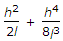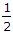# Civil Engineering - Surveying - Discussion

Discussion Forum : Surveying - Section 1 (Q.No. 2)
2.
If h is the difference in level between end points separated by l, then the slope correction is. The second term may be neglected if the value of h in a 20 m distance is less thanm
1 m
2 m
3 m
Explanation:
No answer description is available. Let's discuss.
Discussion:
66 comments Page 1 of 7.

Vijay patel said:   10 years ago
Second term neglect and l = 20.

So h2 = 2L.

h2 = 2*20.

h = 3.3m.

Near equal to 3m.

NamrathaSai said:   9 years ago
Would you please explain the calculations! Why are you taken that h2/2l=1?

Aman said:   9 years ago
If we use any value lesser than 3 and not greater than 3, then answer for slope correction either using the complete formula given above or by eliminating the second term, we will get the same answer. Thus it means that the second term can be eliminated the right answer is 3 and distance kept as 20m.

VIVEK CHANDEL said:   9 years ago
For slopes of 10 percent or less, the correction to be applied to L for a difference d in elevation between tape ends, or for a horizontal offset d between tape ends, may be computed from,

Cs=(h^2)/(2L).

For a slope greater than 10 percent, Cs may be determined from,
Cs={(h^2)/(2L)}+{(h^4)/8(L^3)}.

Nanda said:   9 years ago
@Vijay patel.

h2 = 2*20
h square = 40.
How come h = 3.3?

Rashmi said:   9 years ago
How 3.30?

Ramu said:   9 years ago

From answer verification, If we substitute 1/2 in second term then it will negligible compared to other.

Siva said:   9 years ago
h2 = 40 root 40 = 6.32.

How can you get 3.3?

Raju said:   8 years ago
h square = 40.

Square root of 40 = 6.32 or 6.33.

How get it 3.3?

AKSHAY VASHISHT said:   8 years ago
Second term is neglected for slope less than 10%.

Slope for given problem for:

C) (2/20)*100 = 10%.
D) (3/20)*100 = 15%.

So for h = 2 m or less it is neglected.

And for h = 3 m it will be taken into account.